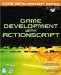# Loops and Iteration

 [ LiB ]

### Loops and Iteration

Loops can be very difficult to understand if you don't pay attention to what they're made out ofconditional statements. Loops are general statements that repeat a block of code if the specified condition is true. If the condition is always true, you'll end up with an infinite loop and your program will never end.

ActionScript has three major kinds of built-in loops (there are others, but they do not concern us now). There is the for loop, which executes a block for a certain number of iterations; the while loop, which executes until a certain condition is met; and a modified version of the while loop, the do while loop, which executes at least once and then terminates when a certain condition is met.

Let's go over a for loop example first. It is the most complicated of all loops because it combines many smaller statements in one place. Let's see the example.

` for (var index = 0; index < 10; index++) {   trace (index); } `

Before breaking into this loop, take a look at the output:

` 0 1 2 3 4 5 6 7 8 9 `

Interesting no? Quite automated if you ask me. You achieved this output with two to three lines of code instead of printing out each number individually. Not bad. So what's really going on here?

The for loop, as I said before, repeats its code block a certain number of times or until a condition is met. Between its parentheses, it contains three statements. The first one initializes a variable, the second one defines the condition that has to be met in order to end the loop, and the third one modifies the variableusually incrementing or decrementing it by the respective operators. Look at the following prototype:

` for (initialize variable; condition; variable modification){ blockOfCode; } `

We followed it exactly in the previous example. In the first part of the for loop, the program initializes the index variable. The second one states, "Execute the block of code while index is less than 10." Meanwhile, the third piece is incrementing index by 1 after every iteration. This causes index to eventually be greater than or equal to 10, making the condition false and therefore ending the loop. In our example, I have included a trace command that outputs what index is during the execution of each iteration, so that you can better understand what's going on.

When would you use such a loop? Let's say you wanted to move a car 10 pixels to the right every frame for 100 pixels. What would you do? You would write a loop that moves the car 10 pixels on every iteration 10 times. Is it sinking in?

But what if you don't know how many times you need to loop? What if you need to loop until a condition is met regardless of the number of times you need to loop? This would be a perfect time to use the while loop. Here's an example.

` var tempVar = 0; while (tempVar < 10) {   trace("Look at me!");   tempVar++; } `

It does exactly how it reads: "while tempVar is less than 10, execute the following block." Notice that here we are modifying the variable in the block instead of the parentheses. If we forget to do this, we will have an infinite loop. Just because it's infinite doesn't mean we can't break out of itall it means is that if we want to get out, we need to use the break command.

` while (1) {   if (1) break; } `

The last example is kind of awkward , isn't it? There are no conditions in any of the parentheses. What I effectively did here is introduce how to use the break command in a loop and demonstrated another way to use a "true" condition to create an infinite state.

Let's step through it. If you remember back when I spoke about Boolean values, I also mentioned that any non-zero value is equivalent to a "true" Boolean value. So guess what? Since this while statement is forever true, it will forever execute.

But wait! There's more! If a condition is always 1, it means it's always true. That means that in this example, that break command will always execute, thus exiting us from that while loophow cool is that?

Now what if you needed the loop to execute at least once before testing any conditions? ActionScript is more than prepared. It has the do while loop at your disposal!

` do {   trace("Print this out"); } while (b < 10); `

So what does this loop do? It enters and executes the block and then tests for a condition. In this case, we will loop while b is less than 10. If you look closely, it will always be 10, because we are not modifying b in any way. Therefore, this will also be an infinite loop.

I gave you some tricky examples in this section, for the sole purpose of developing that sixth sense for programming and error-finding. As we wrap up this looping section, go ahead and loop through it just once more. Doing so will help solidify your knowledge of these wonderful structures. Once you're done, finish up and take a break. You deserve it.

 [ LiB ]Game Development with ActionScript
ISBN: 1592001106
EAN: 2147483647
Year: 2004
Pages: 162
Authors: Lewis Moronta